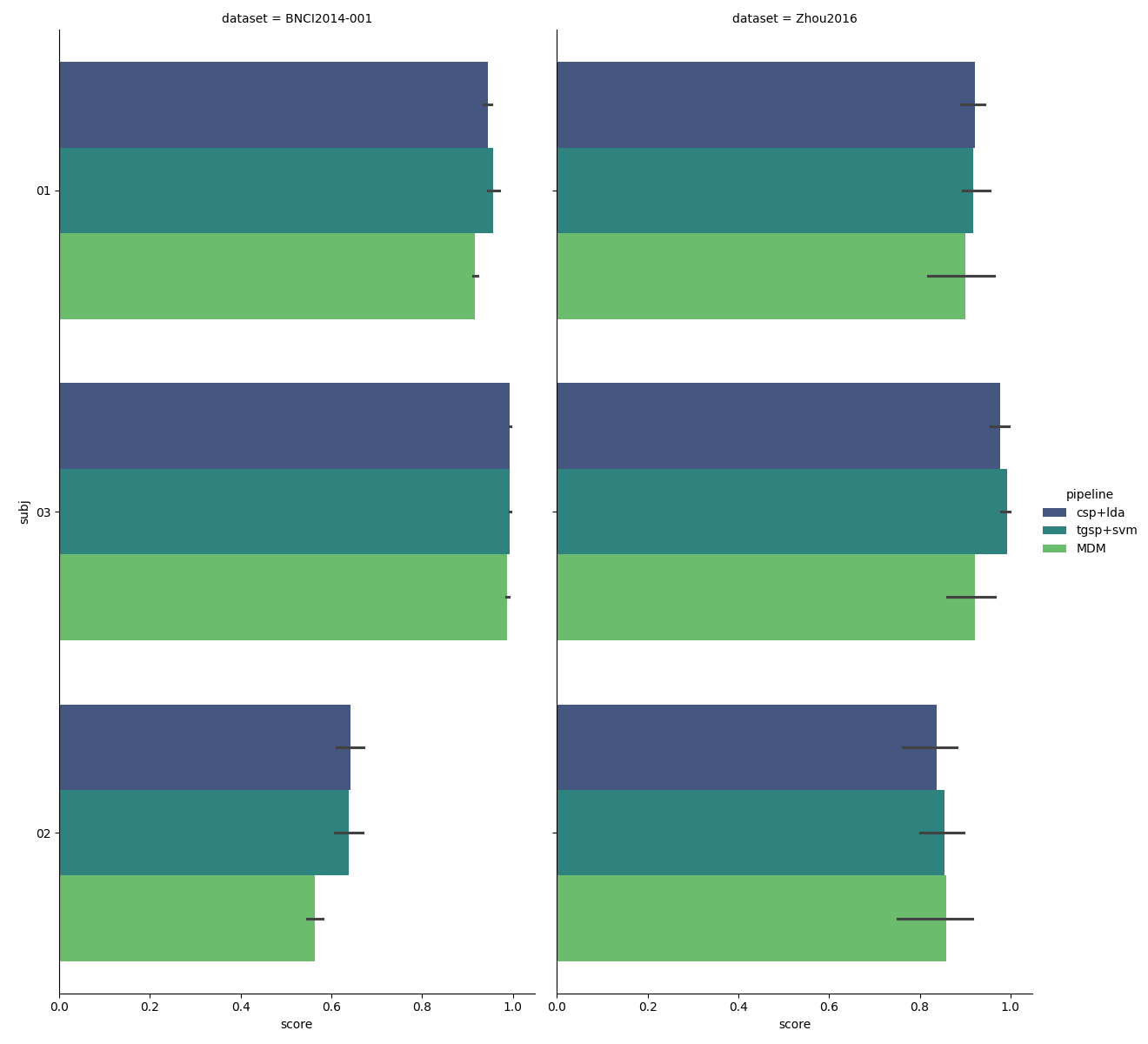# Tutorial 3: Benchmarking mulitple pipelines¶

In this last part, we extend the previous example by assessing the classification score of not one but three classification pipelines.

```# Authors: Pedro L. C. Rodrigues, Sylvain Chevallier
#
# https://github.com/plcrodrigues/Workshop-MOABB-BCI-Graz-2019

import warnings

import matplotlib.pyplot as plt
import mne
import seaborn as sns
from mne.decoding import CSP
from pyriemann.classification import MDM
from pyriemann.estimation import Covariances
from pyriemann.tangentspace import TangentSpace
from sklearn.discriminant_analysis import LinearDiscriminantAnalysis as LDA
from sklearn.pipeline import make_pipeline
from sklearn.svm import SVC

import moabb
from moabb.datasets import BNCI2014001, Zhou2016
from moabb.evaluations import WithinSessionEvaluation

mne.set_log_level("CRITICAL")
moabb.set_log_level("info")
warnings.filterwarnings("ignore")
```

## Creating Pipelines¶

We instantiate the three different classiciation pipelines to be considered in the analysis. The object that gathers each pipeline is a dictionary. The first pipeline is the CSP+LDA that we have seen in the previous parts. The other two pipelines rely on Riemannian geometry, using an SVM classification in the tangent space of the covariance matrices estimated from the EEG or a MDM classifier that works directly on covariance matrices.

```pipelines = {}
pipelines["csp+lda"] = make_pipeline(CSP(n_components=8), LDA())
pipelines["tgsp+svm"] = make_pipeline(
Covariances("oas"), TangentSpace(metric="riemann"), SVC(kernel="linear")
)
pipelines["MDM"] = make_pipeline(Covariances("oas"), MDM(metric="riemann"))
```

The following lines go exactly as in the previous tutorial, where we end up obtaining a pandas dataframe containing the results of the evaluation.

```datasets = [BNCI2014001(), Zhou2016()]
subj = [1, 2, 3]
for d in datasets:
d.subject_list = subj
evaluation = WithinSessionEvaluation(
)
results = evaluation.process(pipelines)
```

Out:

```001-2014-WithinSession:   0%|          | 0/3 [00:00<?, ?it/s]
001-2014-WithinSession:  33%|###3      | 1/3 [00:10<00:21, 10.52s/it]
001-2014-WithinSession:  67%|######6   | 2/3 [00:21<00:10, 10.55s/it]
001-2014-WithinSession: 100%|##########| 3/3 [00:31<00:00, 10.61s/it]
001-2014-WithinSession: 100%|##########| 3/3 [00:31<00:00, 10.59s/it]

Zhou 2016-WithinSession:   0%|          | 0/3 [00:00<?, ?it/s]
Zhou 2016-WithinSession:  33%|###3      | 1/3 [00:08<00:16,  8.33s/it]
Zhou 2016-WithinSession:  67%|######6   | 2/3 [00:15<00:07,  7.53s/it]
Zhou 2016-WithinSession: 100%|##########| 3/3 [00:22<00:00,  7.34s/it]
Zhou 2016-WithinSession: 100%|##########| 3/3 [00:22<00:00,  7.47s/it]
```

As overwrite is set to False, the results from the previous tutorial are reused and only the new pipelines are evaluated. The results from “csp+lda” are not recomputed. The results are saved in ~/mne_data/results if the parameter hdf5_path is not set.

## Plotting Results¶

The following plot shows a comparison of the three classification pipelines for each subject of each dataset.

```results["subj"] = [str(resi).zfill(2) for resi in results["subject"]]
g = sns.catplot(
kind="bar",
x="score",
y="subj",
hue="pipeline",
col="dataset",
height=12,
aspect=0.5,
data=results,
orient="h",
palette="viridis",
)
plt.show()
```Total running time of the script: ( 0 minutes 55.208 seconds)

Gallery generated by Sphinx-Gallery